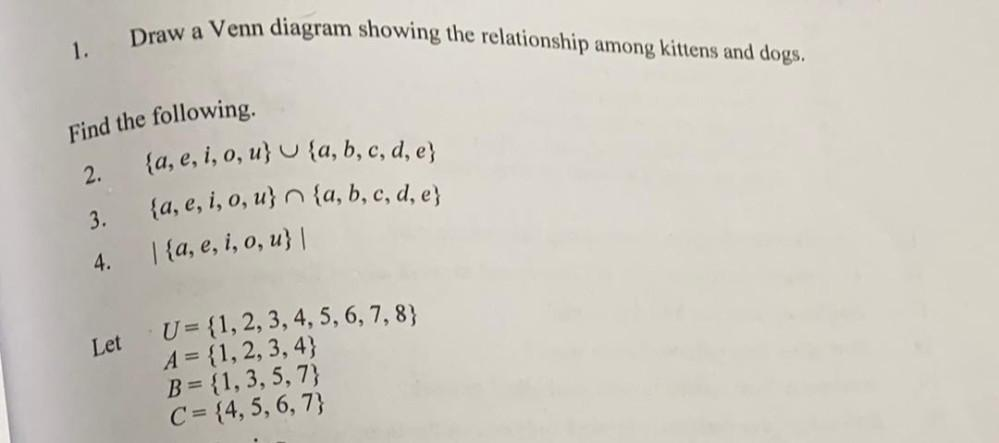Question:

# Draw a Venn diagram showing the relationship among kittens and dogs. 1. Find the following 2. {a, e, i, o, u} U {a, b, c, d, e}Draw a Venn diagram showing the relationship among kittens and dogs. 1. Find the following 2. {a, e, i, o, u} U {a, b, c, d, e} {a, e, i, o, u} n {a, b, c, d, e} 3. 1{a, e, i, o, u} | 4. Let U= {1, 2, 3, 4, 5, 6, 7, 8} A = {1,2,3,4} B = {1,3,5,7) C = {4, 5, 6, 7}